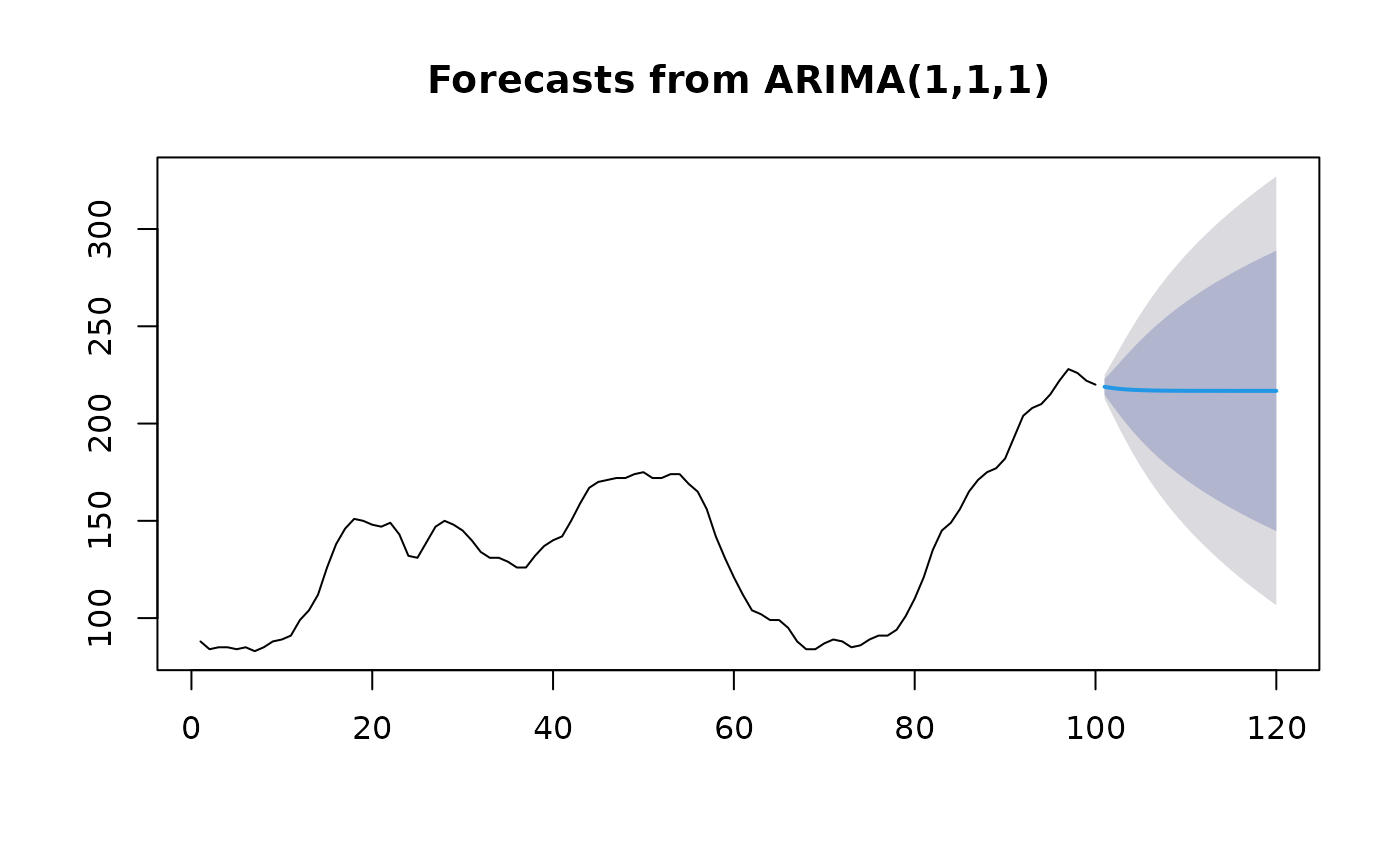Returns best ARIMA model according to either AIC, AICc or BIC value. The function conducts a search over possible model within the order constraints provided.

auto.arima(
y,
d = NA,
D = NA,
max.p = 5,
max.q = 5,
max.P = 2,
max.Q = 2,
max.order = 5,
max.d = 2,
max.D = 1,
start.p = 2,
start.q = 2,
start.P = 1,
start.Q = 1,
stationary = FALSE,
seasonal = TRUE,
ic = c("aicc", "aic", "bic"),
stepwise = TRUE,
nmodels = 94,
trace = FALSE,
approximation = (length(x) > 150 | frequency(x) > 12),
method = NULL,
truncate = NULL,
xreg = NULL,
test.args = list(),
seasonal.test = c("seas", "ocsb", "hegy", "ch"),
seasonal.test.args = list(),
allowdrift = TRUE,
allowmean = TRUE,
lambda = NULL,
parallel = FALSE,
num.cores = 2,
x = y,
...
)

## Arguments

y

a univariate time series

d

Order of first-differencing. If missing, will choose a value based on test.

D

Order of seasonal-differencing. If missing, will choose a value based on season.test.

max.p

Maximum value of p

max.q

Maximum value of q

max.P

Maximum value of P

max.Q

Maximum value of Q

max.order

Maximum value of p+q+P+Q if model selection is not stepwise.

max.d

Maximum number of non-seasonal differences

max.D

Maximum number of seasonal differences

start.p

Starting value of p in stepwise procedure.

start.q

Starting value of q in stepwise procedure.

start.P

Starting value of P in stepwise procedure.

start.Q

Starting value of Q in stepwise procedure.

stationary

If TRUE, restricts search to stationary models.

seasonal

If FALSE, restricts search to non-seasonal models.

ic

Information criterion to be used in model selection.

stepwise

If TRUE, will do stepwise selection (faster). Otherwise, it searches over all models. Non-stepwise selection can be very slow, especially for seasonal models.

nmodels

Maximum number of models considered in the stepwise search.

trace

If TRUE, the list of ARIMA models considered will be reported.

approximation

If TRUE, estimation is via conditional sums of squares and the information criteria used for model selection are approximated. The final model is still computed using maximum likelihood estimation. Approximation should be used for long time series or a high seasonal period to avoid excessive computation times.

method

fitting method: maximum likelihood or minimize conditional sum-of-squares. The default (unless there are missing values) is to use conditional-sum-of-squares to find starting values, then maximum likelihood. Can be abbreviated.

truncate

An integer value indicating how many observations to use in model selection. The last truncate values of the series are used to select a model when truncate is not NULL and approximation=TRUE. All observations are used if either truncate=NULL or approximation=FALSE.

xreg

Optionally, a numerical vector or matrix of external regressors, which must have the same number of rows as y. (It should not be a data frame.)

test

Type of unit root test to use. See ndiffs for details.

test.args

Additional arguments to be passed to the unit root test.

seasonal.test

This determines which method is used to select the number of seasonal differences. The default method is to use a measure of seasonal strength computed from an STL decomposition. Other possibilities involve seasonal unit root tests.

seasonal.test.args

Additional arguments to be passed to the seasonal unit root test. See nsdiffs for details.

allowdrift

If TRUE, models with drift terms are considered.

allowmean

If TRUE, models with a non-zero mean are considered.

lambda

Box-Cox transformation parameter. If lambda="auto", then a transformation is automatically selected using BoxCox.lambda. The transformation is ignored if NULL. Otherwise, data transformed before model is estimated.

Use adjusted back-transformed mean for Box-Cox transformations. If transformed data is used to produce forecasts and fitted values, a regular back transformation will result in median forecasts. If biasadj is TRUE, an adjustment will be made to produce mean forecasts and fitted values.

parallel

If TRUE and stepwise = FALSE, then the specification search is done in parallel. This can give a significant speedup on multicore machines.

num.cores

Allows the user to specify the amount of parallel processes to be used if parallel = TRUE and stepwise = FALSE. If NULL, then the number of logical cores is automatically detected and all available cores are used.

x

Deprecated. Included for backwards compatibility.

...

Additional arguments to be passed to arima.

## Value

Same as for Arima

## Details

The default arguments are designed for rapid estimation of models for many time series. If you are analysing just one time series, and can afford to take some more time, it is recommended that you set stepwise=FALSE and approximation=FALSE.

Non-stepwise selection can be slow, especially for seasonal data. The stepwise algorithm outlined in Hyndman & Khandakar (2008) is used except that the default method for selecting seasonal differences is now based on an estimate of seasonal strength (Wang, Smith & Hyndman, 2006) rather than the Canova-Hansen test. There are also some other minor variations to the algorithm described in Hyndman and Khandakar (2008).

Arima

Rob J Hyndman

## Examples

fit <- auto.arima(WWWusage)
plot(forecast(fit,h=20))